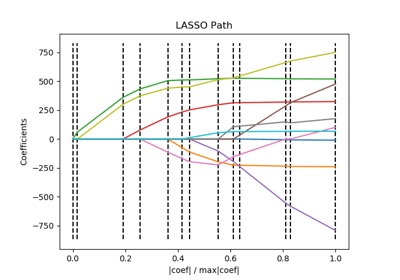/scikit-learn

# sklearn.linear_model.lars_path

`sklearn.linear_model.lars_path(X, y, Xy=None, Gram=None, max_iter=500, alpha_min=0, method=’lar’, copy_X=True, eps=2.220446049250313e-16, copy_Gram=True, verbose=0, return_path=True, return_n_iter=False, positive=False)` [source]

Compute Least Angle Regression or Lasso path using LARS algorithm 

The optimization objective for the case method=’lasso’ is:

```(1 / (2 * n_samples)) * ||y - Xw||^2_2 + alpha * ||w||_1
```

in the case of method=’lars’, the objective function is only known in the form of an implicit equation (see discussion in )

Read more in the User Guide.

Parameters: `X : array, shape: (n_samples, n_features)` Input data. `y : array, shape: (n_samples)` Input targets. `Xy : array-like, shape (n_samples,) or (n_samples, n_targets), optional` Xy = np.dot(X.T, y) that can be precomputed. It is useful only when the Gram matrix is precomputed. `Gram : None, ‘auto’, array, shape: (n_features, n_features), optional` Precomputed Gram matrix (X’ * X), if `'auto'`, the Gram matrix is precomputed from the given X, if there are more samples than features. `max_iter : integer, optional (default=500)` Maximum number of iterations to perform, set to infinity for no limit. `alpha_min : float, optional (default=0)` Minimum correlation along the path. It corresponds to the regularization parameter alpha parameter in the Lasso. `method : {‘lar’, ‘lasso’}, optional (default=’lar’)` Specifies the returned model. Select `'lar'` for Least Angle Regression, `'lasso'` for the Lasso. `copy_X : bool, optional (default=True)` If `False`, `X` is overwritten. `eps : float, optional (default=``np.finfo(np.float).eps``)` The machine-precision regularization in the computation of the Cholesky diagonal factors. Increase this for very ill-conditioned systems. `copy_Gram : bool, optional (default=True)` If `False`, `Gram` is overwritten. `verbose : int (default=0)` Controls output verbosity. `return_path : bool, optional (default=True)` If `return_path==True` returns the entire path, else returns only the last point of the path. `return_n_iter : bool, optional (default=False)` Whether to return the number of iterations. `positive : boolean (default=False)` Restrict coefficients to be >= 0. This option is only allowed with method ‘lasso’. Note that the model coefficients will not converge to the ordinary-least-squares solution for small values of alpha. Only coefficients up to the smallest alpha value (`alphas_[alphas_ > 0.].min()` when fit_path=True) reached by the stepwise Lars-Lasso algorithm are typically in congruence with the solution of the coordinate descent lasso_path function. `alphas : array, shape: [n_alphas + 1]` Maximum of covariances (in absolute value) at each iteration. `n_alphas` is either `max_iter`, `n_features` or the number of nodes in the path with `alpha >= alpha_min`, whichever is smaller. `active : array, shape [n_alphas]` Indices of active variables at the end of the path. `coefs : array, shape (n_features, n_alphas + 1)` Coefficients along the path `n_iter : int` Number of iterations run. Returned only if return_n_iter is set to True.

#### References

  “Least Angle Regression”, Effron et al. http://statweb.stanford.edu/~tibs/ftp/lars.pdf

## Examples using `sklearn.linear_model.lars_path`Lasso path using LARS